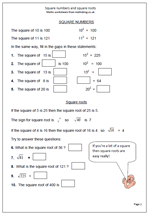# Square numbers and square rootsA square number is a whole number multiplied by itself for example 3 x 3 = 9. This makes 9 a square number. By Year 6 most children will be familiar with square numbers and hopefully will know the first ten or so square numbers off be heart as they all appear in the times tables.

The square root of a number is a value, or number, that can be multiplied by itself to give the original number.

For example: the square root of 16 is 4 because 4 x 4 = 16

The square root of a number is usually written using the ‘tick’ square root sign. Each whole number is the square root of another number:

5 is the square root of 25

6 is the square root of 36 etc

This page takes a quick look at square numbers and square roots and can be found in our Year 6, Knowing Number facts section.

Square numbers and square roots